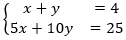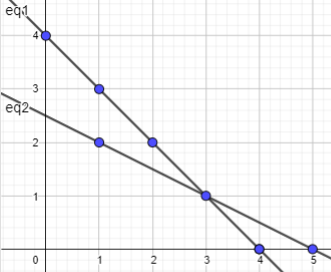# Week 10 Day 1 Lesson Summary

How would you explain "a system of equations" to a classmate who is absent today? What would you say if they asked, "What does it mean to solve the system of equations?"

The graph below represents this system:Which point or points are solutions to the equation x + y = 4?

Which point or points are solutions to the equation 5x + 10y = 25?

Which are solutions to the system?

What are the same and what are different about solving a single linear equation in two variables, say x + y = 4, and solving a system of equations, say